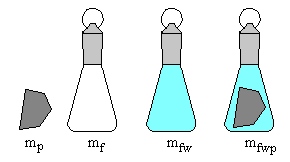# Polymer Chemistry: Polymer Density

•• David Whisnant
• Professor (Chemistry) at Wofford College

Polymer samples usually are irregular in shape, making it difficult to measure their volume directly. There are four routine methods for determining the density of a polymer. One of these methods is called pycnometry. The density can be used to calculate the percent of a polymer that is crystalline.

## Density by Pycnometry

In a pycnometer, the volume of the polymer is determined by the displacement of a liquid or gas. A special container, made of soda glass to avoid static charges, typically is used for the experiment. For our purposes a ground-glass stoppered 25.00-mL Erlenmeyer flask will suffice. We will use water as the liquid.Four measurements are made ($$\pm 0.0001\,g$$):

• mp = mass of polymer
• mf = mass of dry empty flask
• mfw = mass of flask filled with water
• mfwp = mass of flask filled with polymer and water

The temperature of the water also is measured (). The density of water at this temperature can be found in the International Critical Tables and other reference books.

It is important to make sure that all air bubbles have been excluded from the flask when mfw and mfwp are measured. The flask should be filled to the rim and the glass stopper gently dropped into place. It is particularly important to watch for bubbles clinging to the polymer. It is best to use a degassed liquid55, although this may be difficult in our experiments.

The volume of the flask, Vf, is calculated from mf, mfw, and the density of water.

When the polymer is in the flask, the water occupies a smaller volume, Vfp. This volume is calculated from mfwp, mf, mp, and the density of water.

The volume of the polymer is calculated from Vf and Vfp.

Once the volume of the polymer is known, its density can be calculated.

## Percent Crystallinity

We will assume that a semi-crystalline polymer is a simple two-phase system and that the volume of the polymer is a sum of the volumes of the crystalline and amorphous phases.

$V = V_a + V_c$

Let m, ma, and mc be the masses of the polymer, amorphous phase, and crystalline phase. Let D, Da, and Dc be the corresponding densities. Then we can write this equation.

$\dfrac{m}{D}= \dfrac{m_a}{D_a} + \dfrac{m_c}{D_c}$

Divide through by the mass of the polymer.

$\dfrac{1}{D} = \dfrac{m_a/m}{D_a} + \dfrac{m_c/m}{D_c}$

The mass ratios are the percent by mass of the amorphous and crystalline phases, expressed as decimal fractions.

$\dfrac{1}{D} = \dfrac{P_a}{D_a} + \dfrac{P_a}{D_c}$

Assuming that the polymer is a two-phase system, the sum of the percents should be 1.

$\dfrac{1}{D} = \dfrac{1-P_c}{D_a} + \dfrac{P_c}{D_c}$

This equation can be solved for the percent crystallinity:

$P_c = \dfrac{\dfrac{1}{D}-\dfrac{1}{D_a} }{\dfrac{1}{D_c}-\dfrac{1}{D_a} }$

## Amorphous and Crystalline Densities

Determination of the percent crystallinity using densities assumes a knowledge of the densities of 100% amorphous and 100% crystalline polymers. The crystalline density usually is obtained from the unit-cell derived from X-ray diffraction experiments. A reliable value of the amorphous density is much more difficult to obtain55. Often the amorphous density is obtained by extrapolating data taken in the melt, from x-ray scattering, or from the fact that the ratio of the crystalline/amorphous densities is roughly 1.08. Densities of 100% crystalline and 100% amorphous phases are tabulated below for a few common polymers55.

Polymer Da (g/cm3) Dc (g/cm3)
polyethylene 0.853 1.004
poly(vinyl alcohol) 1.291 1.350
poly(vinyl chloride) 1.412 1.477
poly(vinylidene chloride) 1.775 1.957
poly(ethylene terephthalate) 1.336 1.514